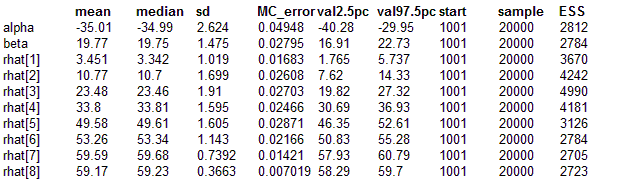#### Beetles: a censoring approach

We return to the Beetles example and in particular the model with the probit link. We exploit
the relation between the probit function and the cumulative normal distribution to reformulate
the model as a censoring problem. To do this we break down each of the binomial observations into a sequence of bernoulli observations. Each bernoulli contributes a factor of Phi(α + βxi) or 1 - Phi(α + βxi) to the likelihood depending on if a one or zero is observed. We then write these factors in terms of a censored observation from a normal with mean equal to α + βxi and precision one.

model
{
for(i in 1 : N) {
for(j in 1 : r[i]) {
y[i, j] ~ dnorm(mu[i], 1)C(0,)
}
for(j in r[i] + 1 : n[i]) {
y[i, j] ~ dnorm(mu[i], 1)C(,0)
}
mu[i] <- alpha.star + beta * (x[i] - mean(x[]))
rhat[i] <- n[i] * phi(mu[i])
}
alpha <- alpha.star - beta * mean(x[])
beta ~ dnorm(0.0,0.001)
alpha.star ~ dnorm(0.0,0.001)
}

##### Data
``` list( x = c(1.6907, 1.7242, 1.7552, 1.7842, 1.8113, 1.8369, 1.8610, 1.8839),      n = c(59, 60, 62, 56, 63, 59, 62, 60),      r = c(6, 13, 18, 28, 52, 53, 61, 60), N = 8) ```
##### Inits for chain 1
``` list(alpha.star=0, beta=0) ```

##### Inits for chain 2
``` list(alpha.star=1, beta=1) ```
ResultsThe estimates agree very well with the direct formulation of the model.

This way of doing probit regression is much slower than the direct method and also leads to MCMC chains with more auto-correlation. However the likelihood for the regression parameters is normal so the method could be combined with reversible jump models which require a normal likelihood.